客服热线：133-4342-757517321150489•••# 冲击试验机 触摸屏冲击试验机 悬臂梁冲击试验机 冲击机

• 面议

CJK-1557

17321150489 021690036278006
• 发货地  上海
• 供货总量  100 台

• 暂无产品目录
 有效期至长期有效 更新时间2020-07-11 04:55 访问次数947
rrrr r r r r r r r r
r r r r r r r r r r r r r r r r r r r r r r r r r r r r r r r r r r r r r r r r
r r r r r r r r r r r r r r
r  r
r r 塑料试验机 塑料拉伸试验机 塑料拉力机 塑料材料试验机r r
r r r r r r r r r
 r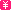r r r 36000.00r r r r 总销量：328 r r
r
r
r r r r r r r r r r r r r r
r  r
r r XJUD-5.5悬臂梁冲击试验机 GB/T1843 冲击试验机悬臂梁 数显r r
r r r r r r r r r
 rr r r 10000.00r r r r 总销量：328 r r
r
r
r r r r r r r r r r r r r r
r  r
r r XNR-400A熔体流动速率仪 塑料熔指试验仪 熔融指数仪 质保一年r r
r r r r r r r r r
 rr r r 5600.00r r r r 总销量：328 r r
r
r
r
r
r r r r r r r r r r r r r r
r  r
r r 热变形、维卡软化点温度测定仪 塑料软化点测定仪 软化点测定仪r r
r r r r r r r r r
 rr r r 35000.00r r r r 总销量：328 r r
r
r
r r r r r r r r r r r r r r
r  r
r r XBM-Ⅱ薄膜拉伸制样机、薄膜取样器、塑料薄膜切样机厂家r r
r r r r r r r r r
 rr r r 25000.00r r r r 总销量：328 r r
r
r
r r r r r r r r r r r r r r
r  r
r r XQZ-I缺口制样机上海供应手动缺口制样机 塑料管材冲击缺口制样机r r
r r r r r r r r r
 rr r r 6000.00r r r r 总销量：328 r r
r
r
r
r
r r r r r r r r r r r r r r
r  r
r r XYZ-70哑铃制样机 上海现货哑铃制样机直销 试样机 哑铃样条制作r r
r r r r r r r r r
 rr r r 6000.00r r r r 总销量：328 r r
r
r
r r r r r r r r r r r r r r
r  r
r r DSC-100 差示扫描量热仪 热分析仪价格 DSC氧化诱导期量热仪r r
r r r r r r r r r
 rr r r 31000.00r r r r 总销量：328 r r
r
r
r r r r r r r r r r r r r r
r  r
r r 排水管材耐压试验机，排水管材静液压试验机，静液压试验机r r
r r r r r r r r r
 rr r r 50000.00r r r r 总销量：328 r r
r
r
r
r
r
r r rr

r
r
r

r

r XJUD-22悬臂梁冲击试验机功能、适用范围： r

r

r 本机主要用于硬质塑料、增强尼龙、玻璃钢、陶瓷、铸石，电绝缘材料等非金属材料冲击韧性的测定。本试验机是化工行业、科研单位、大专院校、质量检测部门、专业生产厂家实验室等单位理想的试验设备。是一种结构简单，操作方便，数据准确可靠的检测仪器。 r

r

r 采用汉字液晶显示，具有能量损失自动修正功能。  r

r

r XJUD-22悬臂梁冲击试验机执行标准： r

r

r 符合ISO180、GB/T1843、GB/T2611、JB/T8761标准的要求。 r

r

r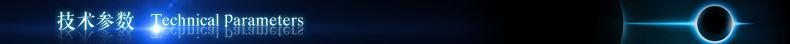r

r

r XJUD-22悬臂梁冲击试验机技术参数： r

r

r     1）冲击速度：3.5m/s r

r

r     2）摆锤能量：11J   22J r

r

r     3）摆锤扬角：150° r

r

r     4）打击中心距：335mm r

r

r     5）测量精度：±0.1%   分辨率：1% r

r

r     6）冲击刀刃至钳口上面距离：22mm±0.2mm r

r

r     7）刀刃圆角半径：R=0.8mm±0.2mm r

r

r     8）能量损失： 11J ≤0.05J ； 22J ≤0.10J r

r

r     9）试样类型：试样为Ⅰ型，为优选型。 r

rr r r r r r r r r r r r r r r r r r r r r r r r r r r r r r r r r
 r r 试样型号 r r r r 长L（mm） r r r r 宽b（mm） r r r r 厚h（m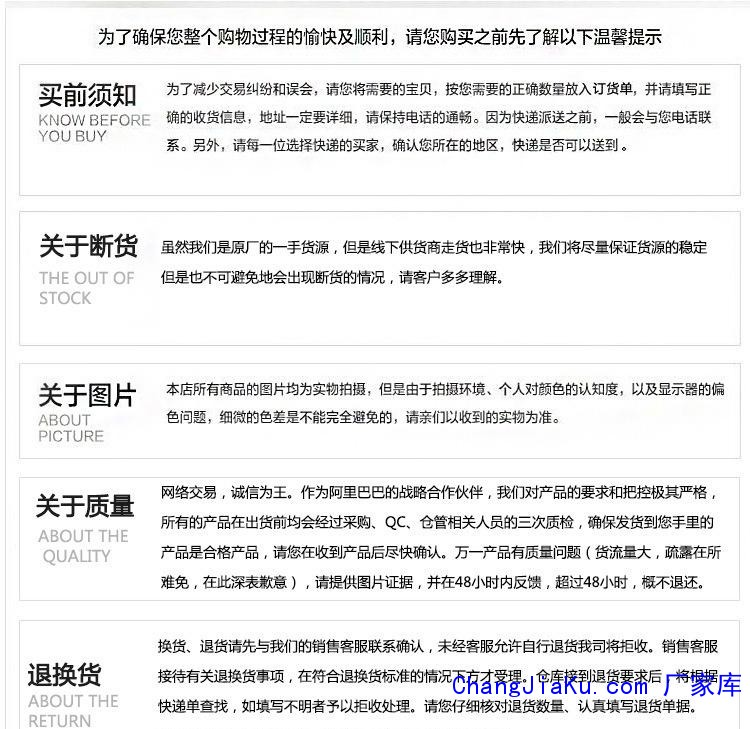m） r r r r 1 r r r r 80±2 r r r r 10.0±0.2 r r r r 4 r r r r 2 r r r r 63.5±2 r r r r 12.7±0.2 r r r r 12.7±0.2 r r r r 3 r r r r 63.5±2 r r r r 12.7±0.2 r r r r 6.4±0.2 r r r r 4 r r r r 63.5±2 r r r r 12.7±0.2 r r r r 3.2±0.2 r r
r

r 10）仪器尺寸：长830mm×宽300mm×高890mm r

r

r 11）所需空间：前后0.4m, 左右1.5m,上部1.5m r

r

r 12）电    源：100VA  220VAC  50HZ  r

r

r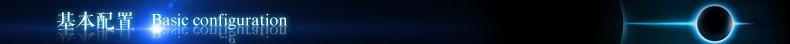r
r
r

r

r XJUD-22悬臂梁冲击试验机配置: r

r

r 1）主机 一台 r

r

r 2）冲击摆 一把 r

r

r 3）砝码 一对（配装在11 J上为22J） r

r

r 4）固定钳口 二个（包括钳口座上一个） r

r

r 5）对中样板 一块 r

r

r 6）内六角搬手4mm、5mm各一个 r

r

r 7）地脚螺钉座 四个 r

r

r 8）电源线 一根 r

r

r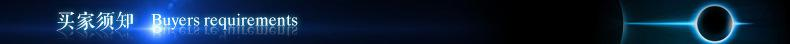r
r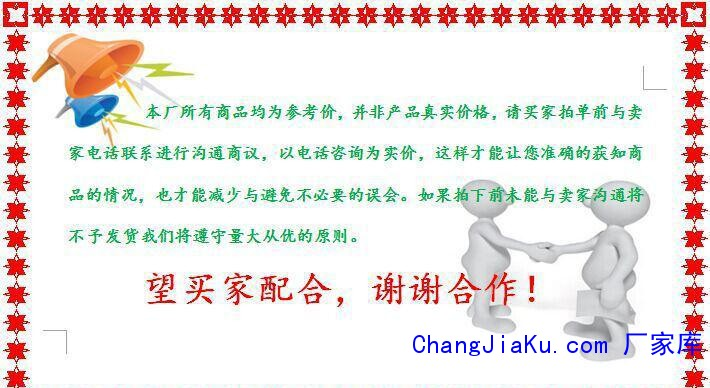r
rr
r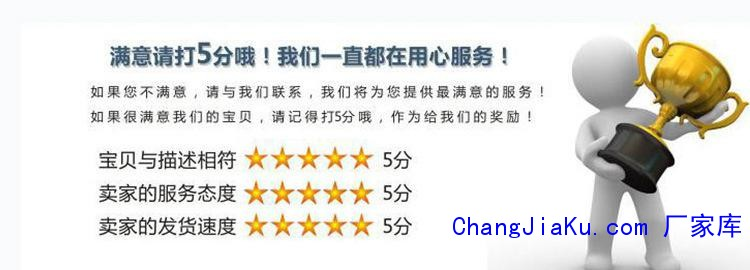r
r
r

r## 纺织物拉力试验机 电线电缆拉力试验机 金## WDW-1M微机控制电子试验机 50KN试验机 拉## XGJ-10A管材静液压试验机 管材耐压爆破试rrrrrrrrrrrrrrrrrrrXGJ-10A系列耐压爆破试验机功能、适用范围：rrrrr静液压试验机适用于各种塑料管材长期静液压试验、管材液压瞬时爆破试验和长期恒定耐压下热塑性管材耐破坏时间的测定。是各科研院所、质检部门及管材生产厂家的检测设备。rrrXGJ-10A系列耐压爆破试验机执行标准： rrr主要技术参数符合rrrGB/T6111《流体输送用热塑性塑料管材耐内压试验方法》rrrGB/T15560《流体输送用塑料管材液压瞬时爆破耐压试验方法》rrrGB/T1

## 管材静液压试验机，管材静液压试验机，管材XGY-10系列管材静液压试验机主要技术参数完全符合GB/T6111、GB/T15560、ISO1167、ISO9080、ASTM?D1598标准的要求，适用于对各种流体输送用塑料管材进行静液压和管材试验。产品具有外型美观、结构简单、精度高、稳定性好、操作方便等特点，是各种研究所质量检测部门及管材生产厂家必备的检测仪器。机型号分类：XGY-10A：LED数字仪表控制、显示试验压力和时间XGY-10B：在仪表控制基础上配PC机（即计算机），采用串口与仪表联接XGY-10

## XWW-20A拉力试验机 PP拉力试验机 电子多功## 管材静液压试验机，管材静液压试验机，上海rrrrrrrXGJ-10管材静液压试验机适用于各种塑料管材长期静液压试验、管材液压瞬时爆破试验和长期恒定耐压下热塑性管材耐破坏时间的测定。是各科研院所、质检部门及管材生产厂家必备的检测设备。rr执行标准：r主要技术参数符合rGB/T6111《流体输送用热塑性塑料管材耐内压试验方法》rGB/T15560《流体输送用塑料管材液压瞬时爆破耐压试验方法》rGB/T18742.1《冷热水用聚丙烯 第二部分：管材》rGB/T13663《给水用聚乙烯PE管材》rGB/T155

## olympus8600塑料测厚仪，壁厚测厚仪，玻璃rrrrrrrrrr8600霍尔效应测厚仪rrrr.称为：复合材料测厚仪，瓶壁测厚仪，塑料瓶测厚仪，霍尔效应测厚仪，壁厚测厚仪。r生产厂家：美国olympus奥林巴斯rMagna-Mike 8600是一款便携式测厚仪，它利用简单的磁法对非铁性材料进行可靠的、重复性极高的厚度测量。Magna-Mike仪器的操作十分简单。测量时，在被测材料的一侧按住或移动磁探头，在材料的另一侧放上或在容器里放入一个小目标钢珠（或目标圆盘、目标钢线）。 探头的霍尔效应传感

## 管材落锤冲击试验机，现货落锤冲击试验机，XJL-300C管材落锤冲击试验机的耐冲击韧性的测定。适用于PVC-U、PVC-C管、PP管、PE管、地下通信管道用塑料管等管材是检测、生产单位、建材行业、科研单位理想的测试仪器。 该试验机具有防止二次冲击功能，采用进口伺服系统，提锤速度快、定位精度好、试验效率高，气阻小、可自动测量高度，落锤提升高度可任意设定，提锤高度自动校准、冲击锤重可通过砝码进行装配、V型铁调整以适应不同管径的试样、锤体下落为自由落体运动，可根据

## wdw-50H电子试验机 0.5级电子试验机 50KN试## HB12-2010卧式投影仪,投影仪，光学检测仪器rrrrr rrrrrrrrrr产品特点：rrrrHB12-2010和HB16-3015卧式投影仪是光、机、电一体化的精密高效测量仪器。它广泛用于机械、模具、仪表、电子、轻工等行业。是计量室和生产车间不可缺少的一种计量检定设备。本仪器能高效率地检测各种形状复杂工件的轮廓尺寸和表面形状，如样板、冲压件、凸轮、螺纹、齿轮、成型铣刀等各种工具、刀具和零件、以及端子、钟表、金刚石等生产厂商。rrrrrrrrrrrrrrrrr的详细资料：rrrrrrrrrr产品特点rr产